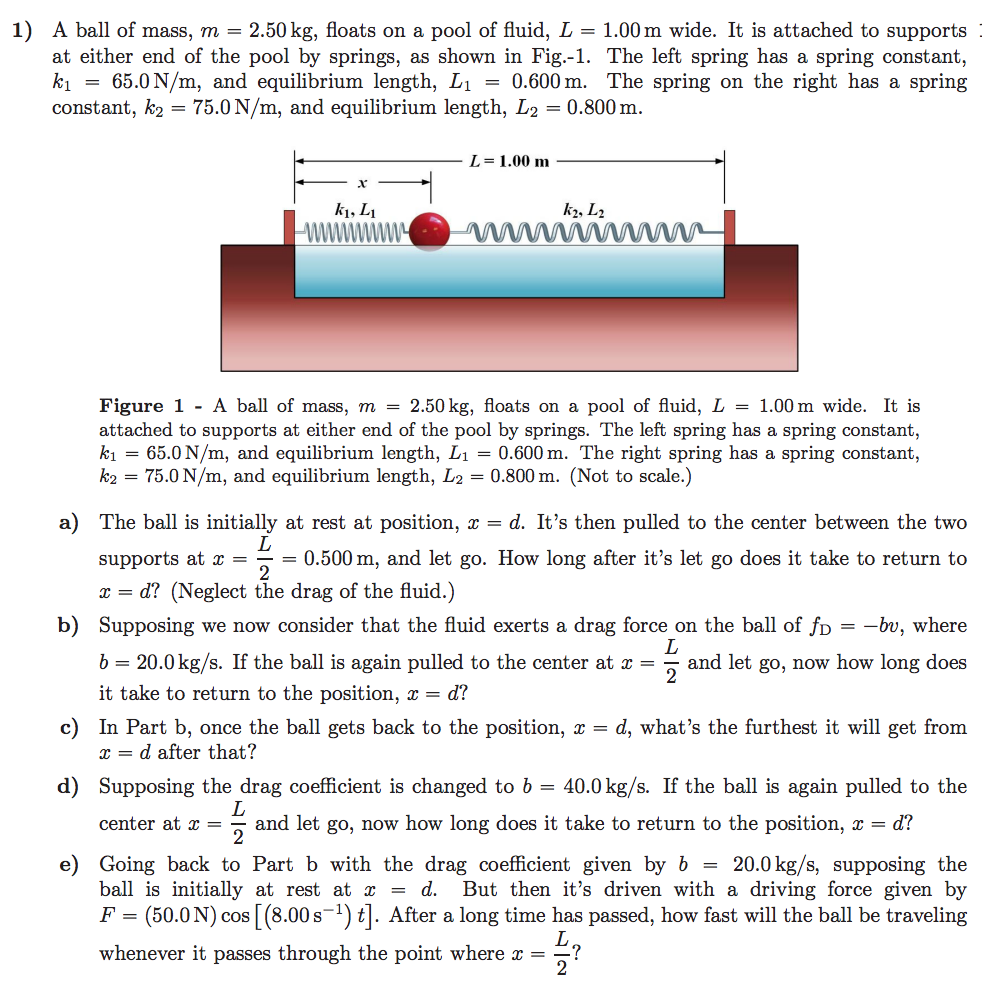# 1) A ball of mass, m = 2.50 kg, floats on a pool of fluid, L-1.00 m wide. It is attached to supports at either end of the pool by springs, as shown in Fig.-1. The left spring has a spring constant, A = 65.0 N/m, and equilibrium length, L1-0.600 m. The spring on the right has a spring constant, k2-75.0N/m, and equilibrium length, L20.800m L = 1.00 m ki, Li Figure 1 -A ball of mass, m = 2.50 kg, floats on a pool of fluid, L = 1.00 m wide. It is attached to supports at either end of the pool by springs. The left spring has a spring constant, A = 65.0 N/m, and equilibrium length, L1 = 0.600 m. The right spring has a spring constant. ka = 75.0 N/m, and equilibrium length, L2 = 0.800 m. (Not to scale.) a) The ball is initially at rest at position, x - d. It's then pulled to the center between the two supports at 0.500 m, and let go. How long after it's let go does it take to return to x- d? (Neglect the drag of the fluid.) Supposing we now consider that the fuld exerts a drag force on the ball of fD b 20.0kg/s. If the ball is again pulled to the center at aand let go, now how long does it take to return to the position, x = d? b) -bu, where c) In Part b, once the ball gets back to the position, x - d, what's the furthest it will get from r-d after that? d) Supposing the drag coefficient is changed to b = 40.0 kg/s. If the ball is again pulled to the center at and let go, now how long does it take to return to the position, x-d? 2 e) Going back to Part b with the drag coefficient given by b -20.0 kg/s, supposing the ball is initially at rest at x - d. But then it's driven with a driving force given by F (50.0N) cos [(8.00s) ]. After a long time has passed, how fast will the ball be traveling whenever it passes through the point where x?

Questionhelp_outlineImage Transcriptionclose1) A ball of mass, m = 2.50 kg, floats on a pool of fluid, L-1.00 m wide. It is attached to supports at either end of the pool by springs, as shown in Fig.-1. The left spring has a spring constant, A = 65.0 N/m, and equilibrium length, L1-0.600 m. The spring on the right has a spring constant, k2-75.0N/m, and equilibrium length, L20.800m L = 1.00 m ki, Li Figure 1 -A ball of mass, m = 2.50 kg, floats on a pool of fluid, L = 1.00 m wide. It is attached to supports at either end of the pool by springs. The left spring has a spring constant, A = 65.0 N/m, and equilibrium length, L1 = 0.600 m. The right spring has a spring constant. ka = 75.0 N/m, and equilibrium length, L2 = 0.800 m. (Not to scale.) a) The ball is initially at rest at position, x - d. It's then pulled to the center between the two supports at 0.500 m, and let go. How long after it's let go does it take to return to x- d? (Neglect the drag of the fluid.) Supposing we now consider that the fuld exerts a drag force on the ball of fD b 20.0kg/s. If the ball is again pulled to the center at aand let go, now how long does it take to return to the position, x = d? b) -bu, where c) In Part b, once the ball gets back to the position, x - d, what's the furthest it will get from r-d after that? d) Supposing the drag coefficient is changed to b = 40.0 kg/s. If the ball is again pulled to the center at and let go, now how long does it take to return to the position, x-d? 2 e) Going back to Part b with the drag coefficient given by b -20.0 kg/s, supposing the ball is initially at rest at x - d. But then it's driven with a driving force given by F (50.0N) cos [(8.00s) ]. After a long time has passed, how fast will the ball be traveling whenever it passes through the point where x? fullscreen